RS Aggarwal Test: Real Numbers - 2

# RS Aggarwal Test: Real Numbers - 2 - Class 10

Test Description

## 15 Questions MCQ Test Mathematics (Maths) Class 10 - RS Aggarwal Test: Real Numbers - 2

RS Aggarwal Test: Real Numbers - 2 for Class 10 2023 is part of Mathematics (Maths) Class 10 preparation. The RS Aggarwal Test: Real Numbers - 2 questions and answers have been prepared according to the Class 10 exam syllabus.The RS Aggarwal Test: Real Numbers - 2 MCQs are made for Class 10 2023 Exam. Find important definitions, questions, notes, meanings, examples, exercises, MCQs and online tests for RS Aggarwal Test: Real Numbers - 2 below.
Solutions of RS Aggarwal Test: Real Numbers - 2 questions in English are available as part of our Mathematics (Maths) Class 10 for Class 10 & RS Aggarwal Test: Real Numbers - 2 solutions in Hindi for Mathematics (Maths) Class 10 course. Download more important topics, notes, lectures and mock test series for Class 10 Exam by signing up for free. Attempt RS Aggarwal Test: Real Numbers - 2 | 15 questions in 20 minutes | Mock test for Class 10 preparation | Free important questions MCQ to study Mathematics (Maths) Class 10 for Class 10 Exam | Download free PDF with solutions
 1 Crore+ students have signed up on EduRev. Have you?
RS Aggarwal Test: Real Numbers - 2 - Question 1

### The decimal expansion of the rational number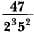will terminate after

Detailed Solution for RS Aggarwal Test: Real Numbers - 2 - Question 1

Multiply by 5 in both numerator and denominator
We get,
(47 × 5)/1000 = 0.235

RS Aggarwal Test: Real Numbers - 2 - Question 2

### Two alarm clocks ring their alarms at regular intervals of 50 seconds and 48 seconds. If they first beep together at 12 noon, at what time will they beep again for the first time?

Detailed Solution for RS Aggarwal Test: Real Numbers - 2 - Question 2

LCM of 50 and 48 = 1200
∴ 1200 sec = 20 min
Hence at 12.20 pm they will beep again for the first time.

RS Aggarwal Test: Real Numbers - 2 - Question 3

### The product of three consecutive integers is divisible by

Detailed Solution for RS Aggarwal Test: Real Numbers - 2 - Question 3

Let the three consecutive positive integers be n, n+1 and n+2.

Whenever a number is divided by 3, the remainder obtained is either 0,1 or 2.

Therefore, n=3p or 3p+1 or 3p+2, where p is some integer.

If n=3p, then n is divisible by 3.

If n=3p+1, then n+2=3p+1+2=3p+3=3(p+1) is divisible by 3.

If n=3p+2, then n+1=3p+2+1=3p+3=3(p+1) is divisible by 3.

So, we can say that one of the numbers among n,n+1 and n+2 is always divisible by 3 that is:

n(n+1)(n+2) is divisible by 3.

Similarly, whenever a number is divided by 2, the remainder obtained is either 0 or 1.

Therefore, n=2q or 2q+1, where q is some integer.

If n=2q, then n and n+2=2q+2=2(q+1) is divisible by 2.

If n=2q+1, then n+1=2q+1+1=2q+2=2(q+1) is divisible by 2.

So, we can say that one of the numbers among n, n+1 and n+2 is always divisible by 2.

Since, n(n+1)(n+2) is divisible by 2 and 3.

Hence, n(n+1)(n+2) is divisible by 6.

RS Aggarwal Test: Real Numbers - 2 - Question 4

If A = 2n + 13, B = n + 7, where n is a natural number, then HCF of A and B is:

Detailed Solution for RS Aggarwal Test: Real Numbers - 2 - Question 4

Taking different values of n we find that A and B are coprime
∴ HCF = 1

RS Aggarwal Test: Real Numbers - 2 - Question 5

The largest number which divides 615 and 963 leaving remainder 6 in each case is

Detailed Solution for RS Aggarwal Test: Real Numbers - 2 - Question 5

we have to  find the largest number which divide 615 and 963 leaving remainder 6 in each case.

so let us subtract 6 from 615 and 963

⇒ 615−6=609

⇒ 963−6=957

now lets find the HCF

⇒ prime factorisation of 609=29×3×3

prime factorisation of 957=29×3×11

now lets take out the common factors from both the cases

⇒ 29 and 3

x=29×3=87

∴ 87 is the number which will divide 615 and 963 leaving remainder 6 in each case.

RS Aggarwal Test: Real Numbers - 2 - Question 6

There are 576 boys and 448 girls in a school that are to be divided into equal sections of either boys or girls alone. The total number of sections thus formed are:

Detailed Solution for RS Aggarwal Test: Real Numbers - 2 - Question 6

HCF of 576 and 448 = 64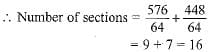RS Aggarwal Test: Real Numbers - 2 - Question 7

The largest number which divides 70 and 125 leaving remainders 5 and 8 respectively is

Detailed Solution for RS Aggarwal Test: Real Numbers - 2 - Question 7

The largest number by which x , y

divisible and gives the remainder a ,

and b is

the HCF of ( x - a ) and ( y - b)
According to the given problem ,

The largest number which divides

70 and 125 leaving remainders 5 and

8 respectively are

HCF of ( 70 - 5 ) = 65 and

( 125 - 8 ) = 117

65 = 5 × 13

117 = 3 × 3 × 13

HCF ( 65 , 117 ) = 13

Required number is 13.

RS Aggarwal Test: Real Numbers - 2 - Question 8

The HCF of 2472, 1284 and a third number N is 12. If their LCM is 23 x 32 x 5 x 103 x 107, then the number N is :

Detailed Solution for RS Aggarwal Test: Real Numbers - 2 - Question 8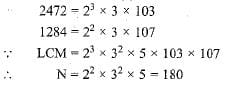RS Aggarwal Test: Real Numbers - 2 - Question 9

The product of a non zero rational and an irrational number is

RS Aggarwal Test: Real Numbers - 2 - Question 10

Two natural numbers whose difference is 66 and the least common multiple is 360, are:

Detailed Solution for RS Aggarwal Test: Real Numbers - 2 - Question 10

Difference of 90 and 24 = 66
and LCM of 90 and 24 = 360
∴ Numbers are 90 and 24.

RS Aggarwal Test: Real Numbers - 2 - Question 11

The decimal expansion of the rational number 14587/1250 will terminate after

RS Aggarwal Test: Real Numbers - 2 - Question 12

Which of the following rational numbers have a terminating decimal expansion?

Detailed Solution for RS Aggarwal Test: Real Numbers - 2 - Question 12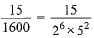The denominator 26 x 52 is of the form 2m x 5n, where m and n are non-negative integers. Hence, it is a terminating decimal expansion.

RS Aggarwal Test: Real Numbers - 2 - Question 13

If two positive integers a and b are written as a = x4 y2 and, b = x2 y3; a, b are prime numbers, then HCF (a, b) is

RS Aggarwal Test: Real Numbers - 2 - Question 14

The LCM of two numbers is 1200. Which of the following cannot be their HCF?

Detailed Solution for RS Aggarwal Test: Real Numbers - 2 - Question 14

The LCM of two numbers = 1200.

By formula, product of two numbers =HCF x LCM

Let the two numbers be y,z

From the details given in question,

HCF x 1200 = y x z

The four options given in question are 600, 400, 500, and 200.

From the available options given, 500 cannot be the HCF since it cannot divide 1200

*Answer can only contain numeric values
RS Aggarwal Test: Real Numbers - 2 - Question 15

Evaluate: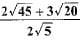Detailed Solution for RS Aggarwal Test: Real Numbers - 2 - Question 15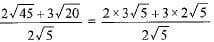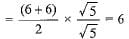## Mathematics (Maths) Class 10

115 videos|478 docs|129 tests
Information about RS Aggarwal Test: Real Numbers - 2 Page
In this test you can find the Exam questions for RS Aggarwal Test: Real Numbers - 2 solved & explained in the simplest way possible. Besides giving Questions and answers for RS Aggarwal Test: Real Numbers - 2, EduRev gives you an ample number of Online tests for practice

## Mathematics (Maths) Class 10

115 videos|478 docs|129 tests